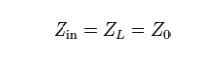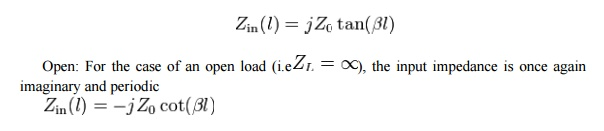## Chapter: Transmission Lines and Waveguides : Transmission Line Theory

In ordinary telephone cables, the wires are insulated with paper and twisted in pairs, therefore there will not be flux linkage between the wires, which results in negligible inductance, and conductance.

In ordinary telephone cables, the wires are insulated with paper and twisted in pairs, therefore there will not be flux linkage between the wires, which results in negligible inductance, and conductance. If this is the case, there occurs frequency and phase distortion in the line.

## Quarter wave length

For the case where the length of the line is one quarter wavelength long, or an odd multiple of a quarter wavelength long, the input impedance becomesAnother special case is when the load impedance is equal to the characteristic impedance of the line (i.e. the line is matched), in which case the impedance reduces to the characteristic impedance of the line so thatFor all l and all λ.

Short : For the case of a shorted load (i.e. ZL = 0), the input impedance is purely imaginary and a periodic function of position and wavelength (frequency)Study Material, Lecturing Notes, Assignment, Reference, Wiki description explanation, brief detail